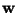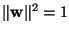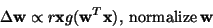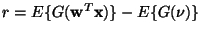Next: Other neural (adaptive) algorithms Up: Algorithms for ICA Previous: Non-linear PCA algorithms

## Neural one-unit learning rules

Using the principle of stochastic gradient descent, one can derive simple algorithms from the one-unit contrast functions explained above. Let us consider first whitened data. For example, taking the instantaneous gradient of the generalized contrast function in (28) with respect to, and taking the normalizationinto account, one obtains the following Hebbian-like learning rule(40)

where the constant may be defined, e.g. as. The nonlinearity g can thus be almost any nonlinear function; the important point is to estimate the multiplicative constant r in a suitable manner [73,65]. In fact, it is enough to estimate the sign of r correctly, as shown in . Such one-unit algorithms were first introduced in  using kurtosis, which corresponds to taking g(u)=u3. Algorithms for non-whitened data were introduced in [71,103].

To estimate several independent components, one needs a system of several units, each of which learns according to a one-unit learning rule. The system must also contain some feedback mechanisms between the units, see e.g. [71,73]. In , a special kind of feedback was developed to solve some problems of non-locality encountered with the other learning rules.Next: Other neural (adaptive) algorithms Up: Algorithms for ICA Previous: Non-linear PCA algorithms
Aapo Hyvarinen
1999-04-23